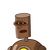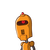# 14. The sum of all the probabilities is(a) 0(b) 1(c) 2(d) none​

14. The sum of all the probabilities is
(a) 0
(b) 1
(c) 2
(d) none​

### 2 thoughts on “14. The sum of all the probabilities is<br />(a) 0<br />(b) 1<br />(c) 2<br />(d) none​”

1.Step-by-step explanation: option (b)

1 is the answer for above question

2.Sum of all probability is (b) 1

Ex:Like probability of some event to happen

1/2

probability of event not to happen = 1/2

So sum of these probability =1/2+1/2=

2/2=1